# Tough GMAT Problem Solving Questions

Must solve GMAT Problem Solving practice questions. The categorization of the topic in which the question is selected from and the concept tested are listed in the right column for each question. For each question detailed explanatory answers, solution videos and slide decks are provided. The questions cover all areas tested in the GMAT quant sections viz., algebra, arithmetic, geometry and word problems.

• 1
• If x is a positive integer such that (x-1)(x-3)(x-5)....(x-93) < 0, how many values can x take? A. 47
B. 23
C. 46
D. 21
E. 22
• Algebra / Inequalities
• Positive & Negative Numbers
• Odd & Numbers
x can take 23 integer values.

Solution

• 2
• If two distinct integers a and b are picked from {1,2,3,4....100} and multiplied, what is the probability that the resulting number has EXACTLY 3 factors? A.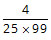B.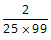C.D.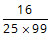E.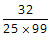Solution

• 3
• Working alone, A can complete a task in ‘a’ days and B in ‘b’ days. They take turns in doing the task with each working 2 days at a time. If A starts they finish the task in exactly 10 days. If B starts, they take half a day more. How long does it take to complete the task if they both work together? A.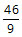B.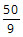C.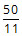D.E.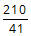• Algebra
• Word Problems
• Work Time

Working together they will takedays.

Solution

• 4
• In the figure given below, ABC and CDE are two identical semi-circles of radius 2 units. B and D are the mid points of the arc ABC and CDE respectively. What is the area of the shaded region?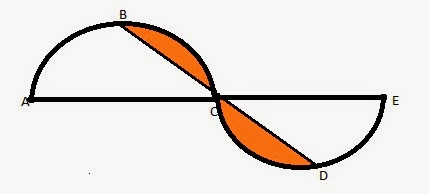A. 4π - 1
B. 3π - 1
C. 2π - 4
D. ½(3π - 1)
E. 2π - 2
• Geometry
• Semicircles
• Isosceles Triangles
The area of the shaded region is
(2π - 4) sq units.

Solution

• 5
• If a, b, and c are not equal to zero, what is the difference between the maximum and minimum value of S? $$S = 1+\frac { \left| a \right| }{ a } +\frac {2 \left| b \right| }{ b } +\frac {3 \left| ab \right| }{ ab } -\frac {4 \left| c \right| }{ c } \\$ A. 12 B. 14 C. 22 D. 20 E. 18 • Algebra • Absolute Value • Correct AnswerChoice$E).
The difference between the maximum and minimum value is 18.

Solution

• 6
• Consider a set S = {2, 4, 6, 8, x, y} with distinct elements. If x and y are both prime numbers and 0 < x < 40 and 0 < y < 40, which of the following MUST be true? I. The maximum possible range of the set is greater than 33.
II. The median can never be an even number.
III. If y = 37, the average of the set will be greater than the median.

A. I only
B. I and II only
C. I and III only
D. III only
E. I, II, and III
• Statistics
• Arithmetic
• Range, Mean, Median
All 3 statements are correct.

Solution

• 7
• If x and y are integers and |x - y| = 12, what is the minimum possible value of xy?
A. -12
B. -18
C. -24
D. -36
E. -48
• Algebra
• Absolute Value
The minimum possible value for xy is -36.

Solution

• 8
• Three positive integers a, b, and c are such that their average is 20 and a ≤ b ≤ c. If the median is (a + 11), what is the least possible value of c? A. 23
B. 21
C. 25
D. 26
E. 24
• Statistics
• Arithmetic
• Mean & Median